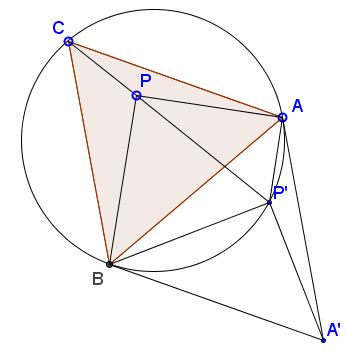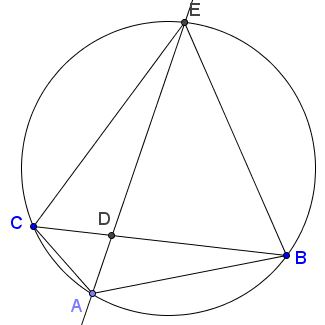Van Schooten's and Pompeiu's TheoremsWhat are these? A Mathematical Droodle

3 November 2014, Created with GeoGebra

ExplanationVan Schooten's and Pompeiu's Theorems

The applet illustrates two theorems discovered three hundred years apart. Let $P$ be a point in the plane of an equilateral $\Delta ABC.$ Both theorems are concerned with the relative lengths of the segments $AP,$ $BP,$ and $CP,$ joining $P$ the vertices of $\Delta ABC.$

Theorem (F. van Schooten, 1615 - 1660)

For $P$ on the circumcircle of $\Delta ABC.$ The longest of the three segments equals the sum of the shorter two.

Theorem (D. Pompeiu, 1873 - 1954)

For $P$ not on the circumcircle of $\Delta ABC,$ there exists a triangle with sides $PA,$ $PB,$ and $PC.$

(The triangle in question is known as Pompeiu's triangle.)Ptolemy's theorem offers one approach to proving the theorems. A proof below is more elementary.

For a proof, rotate the plane $60^{\circ}$ around $B$ so as to make vertex $C$ overlap vertex $A.$ Let $A'$ and $P'$ be the images of $A$ and $P,$ respectively. I'll show that $APP'$ is Pompeiu's triangle.

By the construction, $\Delta BPP'$ is equilateral, so that $PP' = BP.$ In addition, $AP'$ is the image under the above rotation of $CP,$ so that $AP' = CP.$ The remaining side in $\Delta APP'$ is just $AP,$ and we are done with Pompeiu's theorem.

Assuming $A,$ $P,$ and $P'$ are collinear (i.e., when Pompeiu's triangle is degenerate), $\angle AP'B$ is either $60^{\circ}$ or $120^{\circ}.$ (This is because it is either equal to $\angle BPP',$ which is $60^{\circ},$ or is supplementary to it.) But then the quadrilateral $ABCP$ is cyclic, proving van Schooten's theorem and its converse.

Remark 1

Van Schooten's configuration appears in the following simple lemma:

Assume in $\Delta ABC$ $\angle BAC=120^{\circ}.$ Let $D$ be the foot of the angle bisector from $A.$ Then $\displaystyle AD=\frac{AB\cdot AC}{AB+AC}.$

For a proof, consider the circumcircle $(ABC)$ and let $E$ be the point where $AD$ crosses $(ABC).$ $E$ is the midpoint of the arc $BC$ opposite $A.$ This makes $\Delta BCE$ equilateral and the required proportion could be written as $\displaystyle AD=\frac{AB\cdot AC}{AE}$ (or, in the notations used for Pompeiu's theorem, $\displaystyle DP'=\frac{AP'\cdot BP'}{CP'},$ where $D$ is the intersection of $CP'$ and $AB.)$Triangles $CDE$ and $ADB$ are similar, so that $\displaystyle\frac{AD}{CD}=\frac{AB}{CE}.$ But $CE=BC,$ hence,

$\displaystyle AD=AB\frac{CD}{BC}.$

By a property of angle bisectors, $\displaystyle\frac{AB}{AC}=\frac{BD}{CD},$ implying

$\displaystyle\frac{AB+AC}{AC}=\frac{BD+CD}{CD}=\frac{BC}{CD}.$

Combining the two ratios yields $\displaystyle AD=AB\frac{AC}{AB+AC}=\frac{AB\cdot AC}{AB+AC}.$

Remark 2

van Schooten's theorem admits a non-trivial extension for triangles that are not equilateral!

(There is another proof.)

There is also a converse construction of finding an eqilateral triangle.

References

1. T. Andreescu, R. Gelca, Mathematical Olympiad Challenges, Birkhäuser, 2004, p. 4.Ptolemy's Theorem